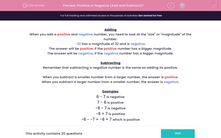# Positive or Negative (Add and Subtract)?

In this worksheet, students add and subtract positive and number answers to decide whether the answer is positive or negative.This content is premium and exclusive to EdPlace subscribers.Key stage:  KS 2

Curriculum topic:   Maths and Numerical Reasoning

Curriculum subtopic:   Mixed Problems

Difficulty level:#### Worksheet Overview

When you add a positive and negative number, you need to look at the "size" or "magnitude" of the number.

-32 has a magnitude of 32 and is negative.

The answer will be positive, if the positive number has a bigger magnitude.

The answer will be negative, if the negative number has a bigger magnitude.

Subtracting

Remember that subtracting a negative number is the same as adding its positive.

When you subtract a smaller number from a larger number, the answer is positive.

When you subtract a larger number from a smaller number, the answer is negative.

Examples

6 - 7 is negative

7 - 6 is positive

-6 - 7 is negative

-6 + 7 is positive

-6 - -7 = -6 + 7 which is positive

### What is EdPlace?

We're your National Curriculum aligned online education content provider helping each child succeed in English, maths and science from year 1 to GCSE. With an EdPlace account you’ll be able to track and measure progress, helping each child achieve their best. We build confidence and attainment by personalising each child’s learning at a level that suits them.

Get started